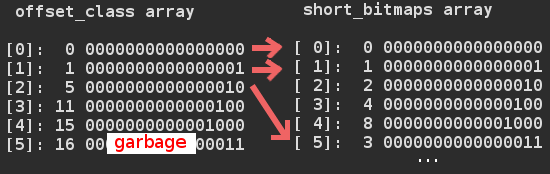# How do succinct data types work in libcds? – Part II

## How does RRR work?

todo

#### Building the RRR structure

When you build an RRR structure you have to define the bitstring and a sample rate.

`BitSequenceRRR::BitSequenceRRR(const BitString & bs, uint sample_rate);`

bs is the source data
sample_rate is todo

BLOCK_SIZE := 15, by definition.
C_field_bits := 4, it means how many bits required to express BLOCK_SIZE

Note: I use these values from here without the variable names.

C_len := number of 4 bit blocks in source data
C := uint array with size enough to hold C_len * 4 bits

It makes a table_offset object named “E” with BLOCK_SIZE parameter:

`table_offset::table_offset(uint u)`

This call creates:

• 2 simple arrays: short_bitmaps[2^(15+1)], offset_class[15+2];
• 2 two dimensional arrays (with dimension (15+1)x(15+1)): binomial, log2binomial
• 1 array (_List) with size 2^(15+1+1)

See how these tables look like: binomial table, log2binomial table, short_bitmaps, offset_class
You get the log2binomial table by ceiling the log2 values of the binomial table.
You get short_bitmaps array by iterating all possible numbers with the given number of 1s in it’s binary form.
offset_class array contains the offsets (in short_bitmaps) of the classes of ones.Note: short_bitmaps and offset_class tables was generated with BLOCK_SIZE set to 4 for readability.

I have no idea what is the purpose of _List after the creation of these tables (it’s saved as rev_offset). The algorithm for filling sort_bitmaps and offset_class arrays are REALLY complicated, contains deep recursion, names written in Spanish.. so I skip describing it’s inner working.

todo

It iterates through the source data and counts the number of ones in 15 bit groups (see BLOCK_SIZE). In the iteration it always sets the next 4 bits (see C_field_bits) of array “C” with this number.

todo

While iterating the total number of ones is summed and … todo

Getting the 15 bit parts and setting the 4 bit parts in uint arrays is done by using the uint arrays as packed arrays.

Note:
These are the functions for handling arrays as packed arrays (libcdsBasics.h):

```inline uint get_var_field(const uint *A, const size_t ini, const size_t fin);
inline void set_field(uint *A, const size_t len, const size_t index, const uint x)
```

todo

#### Problems and inefficiencies in RRR

• Zeroing is done one by one. A standard library call should be used instead!
• generaClase function uses deep recursion which is inefficient
• There are lots of +1s,+2s in array dimensions which are unnecessary
• todo